# Cylindrical Coil SpringThis test lets you check the rigidity of a cylindrical coil spring clamped at an extremity and submitted to a longitudinal force. You will use 1D and 3D models.Reference:

Analytical solution

##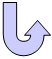Specifications

### Geometry Specifications

 Spring height: h = 40 mmCoiling diameter: D = 40 mmWire diameter: d = 0.9 mmNumber of turns: i = 8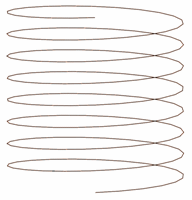### Analysis Specifications

 Young Modulus (material): E = 70 GPa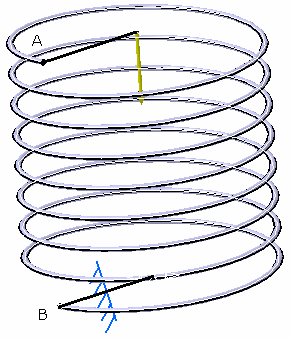Poisson's Ratio (material): ν = 0.346 Mesh Specifications: 1D mesh: beam element of 1 mm 3D mesh: Linear hexahedron elements (HE8): sweep 3D from linear quadrangle elements (QD4) of 0.1 mm. Restraints: Clamp on B Loads: Distributed force in compression at point A Fz = -10 mN

##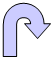Results

### Analytical solution

For an acute angle of inclination of turns (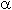< 8° ) the spring stiffness is: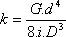Where:
G: shear modulus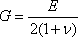For= 7.13°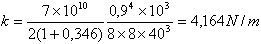### Computed results

You may get the same stiffness as previously using the formula: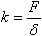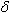: spring displacement

The table below presents the analysis results:

 Models Maximum displacement on z [mm] Experimental k [N/m] Normalized results 1D 2.399 4.168404 1.001058 3D 2.52412 3.961777 0.951435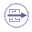To Perform the Test:

The spring_1d.CATAnalysis document presents a complete analysis of this case.

The spring_3d.CATAnalysis document presents a complete analysis of this case.

To compute the case, proceed as follow:

1. Open the CATAnalysis document.

2. Compute the case in the Generative Structural Analysis workbench.

3. Create a global sensor (Maximum displacement) and a local sensor (Displacement vector).